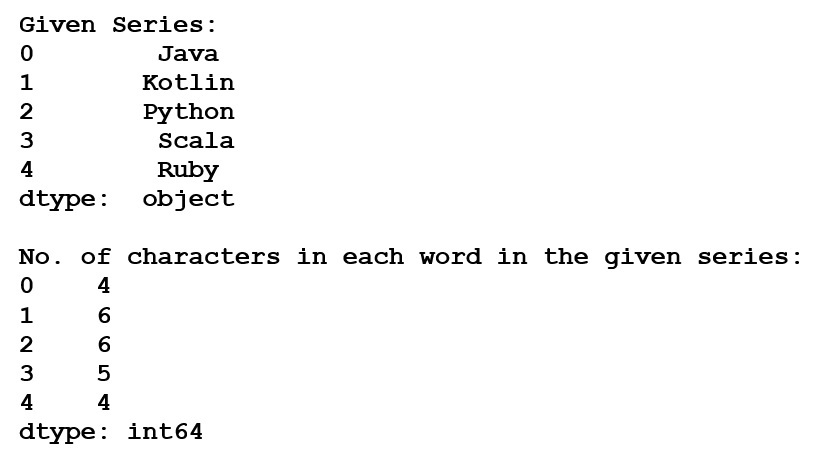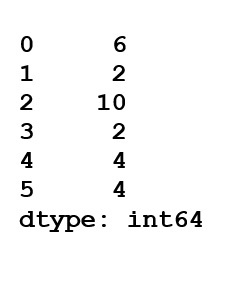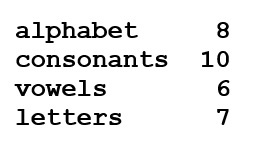# Calculate the number of characters in each word in a Pandas series

To calculate the numbers of characters we use Series.str.len(). This function returns the count of the characters in each word in a series.

Syntax: Series.str.len()

Return type: Series of integer values. NULL values might be present too depending upon caller series.

Another way to find the number of characters by using len() function (that wrapped inside the map function) so that the given series substitutes the value of length by taking series of data as input using pandas.series().

Let’s see some examples:

Example 1: We take input of words and count each character of words with the help of Series.map() that substitute the values and gives output using a function named as calc.

 `# Import pandas library ` `import` `pandas as pd ` ` `  `# Input series of words ` `words ``=` `pd.Series([``'Java'``, ``'Kotlin'``, ` `                   ``'Python'``, ``'Scala'``, ` `                    ``'Ruby'``]) ` `print``(``"Given Series:"``) ` `print``(words) ` ` `  `# Substituting values using map ` `rst ``=` `words.``map``(``lambda` `calc: ``len``(calc)) ` `print``(``"No. of characters in each word in the given series:"``) ` `print``(rst) `

Output:Example 2: We input a series of words and substitute values using Series.map() and use Series.apply() for single values.

 `# Import pandas library ` `import` `pandas as pd ` ` `  `# Input series of words ` `words ``=` `pd.Series([``'Number'``, ``'Of'``, ``'Characters'``, ` `                   ``'In'``, ``'Each'``, ``'Word'``])[``len``(count) ` `                   ``for` `count ``in` `words] ` ` `  `# Substituting values using a map ` `words.``map``(``len``) ` ` `  `# For single values ` `words.``apply``(``len``) `

Output:Example 3: To print in a specific format of word with its length.

 `# Import pandas library ` `import` `pandas as pd ` ` `  `# Input series of words ` `words ``=` `pd.Series([``'alphabet'``, ``'consonants'``,  ` `                   ``'vowels'``, ``'letters'``]) ` ` `  `# Display length of words ` `# along with words ` `for` `i ``in` `range``(``len``(words)): ` `    ``print``(words[i], words.``str``.``len``()[i])      `

Output:My Personal Notes arrow_drop_upCheck out this Author's contributed articles.

If you like GeeksforGeeks and would like to contribute, you can also write an article using contribute.geeksforgeeks.org or mail your article to contribute@geeksforgeeks.org. See your article appearing on the GeeksforGeeks main page and help other Geeks.

Please Improve this article if you find anything incorrect by clicking on the "Improve Article" button below.

Article Tags :

Be the First to upvote.

Please write to us at contribute@geeksforgeeks.org to report any issue with the above content.